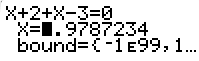## Solve w/Graphing Calulator

Solve
Graphically
w/Calculator: with TI82,   with TI83,   TI85 or TI86,   TI89
Some Sample Problems

 Solve the equation x + 2 = - x + 3 with a TI82 1st: Rewrite the equations so one side or member is 0. 2nd: Press [MATH] then [0:Solver(] 3rd: Enter the expression in step 1 as part of the argument of the Solver function.     Also enter the variable, x, and     a constant or seed value or guess to start the computation.     Ex. Solve(expression, variable, seed     Ex. Solve(x + 2 + x - 3, x, 5 4th: Press [ENTER] to solve.   Solve the equation x + 2 = - x + 3 with a TI83 1st: Rewrite the equations so one side or member is 0. 2nd: Press [MATH] then [0:Solver(] 3rd: Press the curser to get up to the equation line. 4th: Type the expression from step 1 after the =.5th: Press the curser down to the line with the variable you wish to find.6th: Press [SOLVE], which is [ALPHA][ENTER], to solve. Solve the equation x² + 3x = 9 with a TI84 1st: Rewrite the equations so one side or member is 0. 2nd: Press [MATH] then [0:Solver(] 3rd: Press the curser to get up to the equation line. 4th: Type the expression from step 1 after the =. Click on image to enlarge.5th: Press the curser down to the line with the variable you wish to find. 6th: Press [SOLVE], which is [ALPHA][ENTER], to solve. Solve the equation x + 2 = - x + 3 with a TI85 or TI86 1st: Press SOLVER (above GRAPH) 2nd: Enter the equation     Ex. equ:leftside = rightside     Ex. equ:x + 2 = - x + 3 (The = is above [STO>]. 3rd: Press [ENTER] 4th: Be sure the curser is on the line with the varible you want. 5th: On the soft keypad, press [SOLVE].  Solve on the calculator using POLY (85, 86). 1st: Press POLY (above PRGM) 2nd: Enter order 2 for 2nd degree, 3 for 3rd degree, ... 3rd: Enter each coefficient as pictured in the equation. 4th: On the soft keypad, press [SOLVE].  Solve the equation x + 2 = - x + 3 with a TI89 1st: Press [F2], Algebra 2nd: Press [Enter] for Solve( 3rd: Continue typing on the command line:     Ex. solve(x+2=-x+3,x) entering the equation, a comma, then the variable, and a ) to end the arguement of the solve function 4th: Press [ENTER].  Solve these problems with calculator and check the answer. 1.)     x + 2 = - x + 3   Answers x = 1/2 2.)     x + 5 = 5 + x   Answers all numbers -- an identity 3.)     x + 5 = 6   Answers x = 1, conditional 4.)     x + 5 = 7 + x   Answers no solution, a contradiction  Solve and check the first 4 before solving these. 5.)     x + 2 = 0   Answers x = - 2 6.)     x² - 5x - 14 = 0   Answers x = -2 or x = 7© 2007, 2012, 2017 A. Azzolino www.mathnstuff.com/math/spoken/here/2class/400/solve.htm